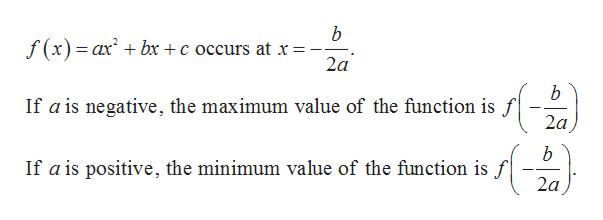# What is the maximum value of the function f(x) = −x2 + 2x + 4? (Enter an exact number.)

Question
1 views

What is the maximum value of the function f(x) = −x2 + 2x + 4? (Enter an exact number.)

check_circle

Step 1

Given,

Step 2

The maximum or minimum value o...help_outlineImage Transcriptioncloseb f(x)ax bx +c occurs at x = 2a b If a is negative, the maximum value of the function is f 2a b If a is positive, the minimum value of the function is f 2a fullscreen

### Want to see the full answer?

See Solution

#### Want to see this answer and more?

Solutions are written by subject experts who are available 24/7. Questions are typically answered within 1 hour.*

See Solution
*Response times may vary by subject and question.
Tagged in

### Algebra